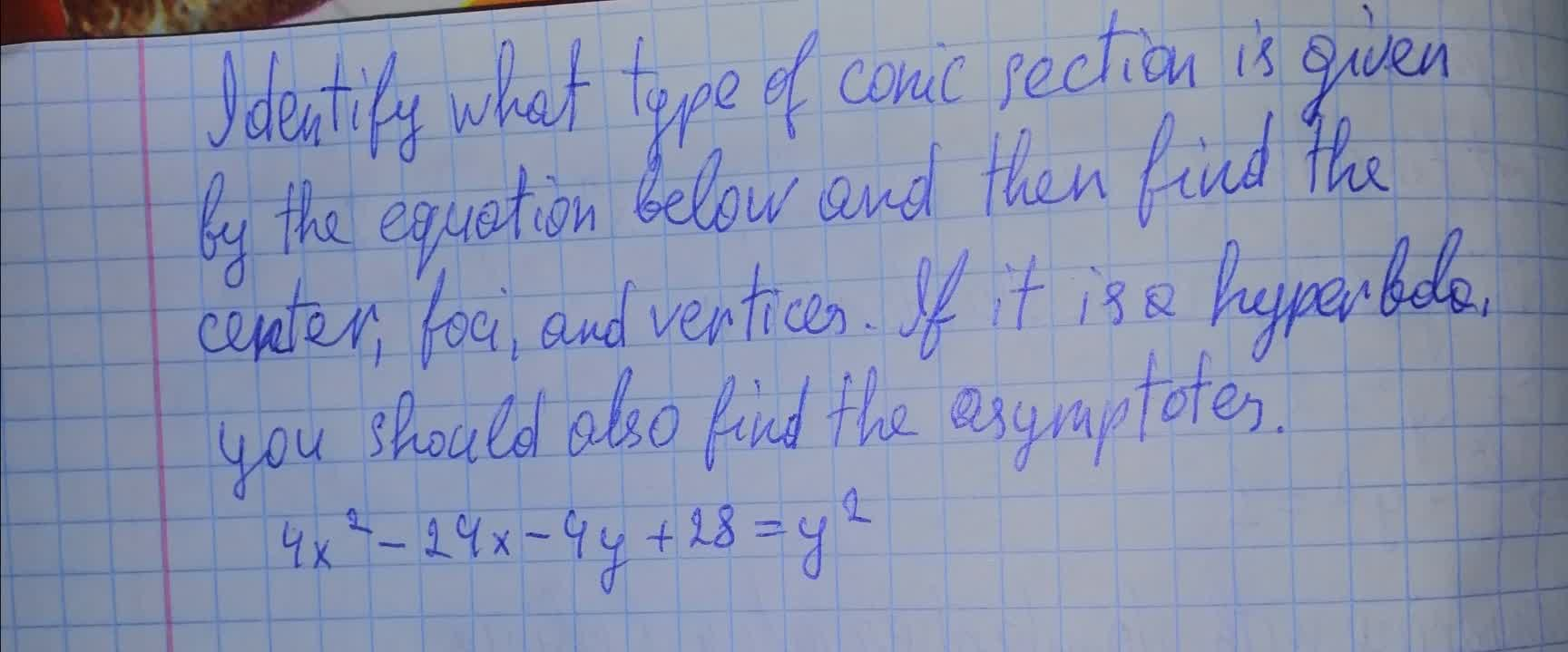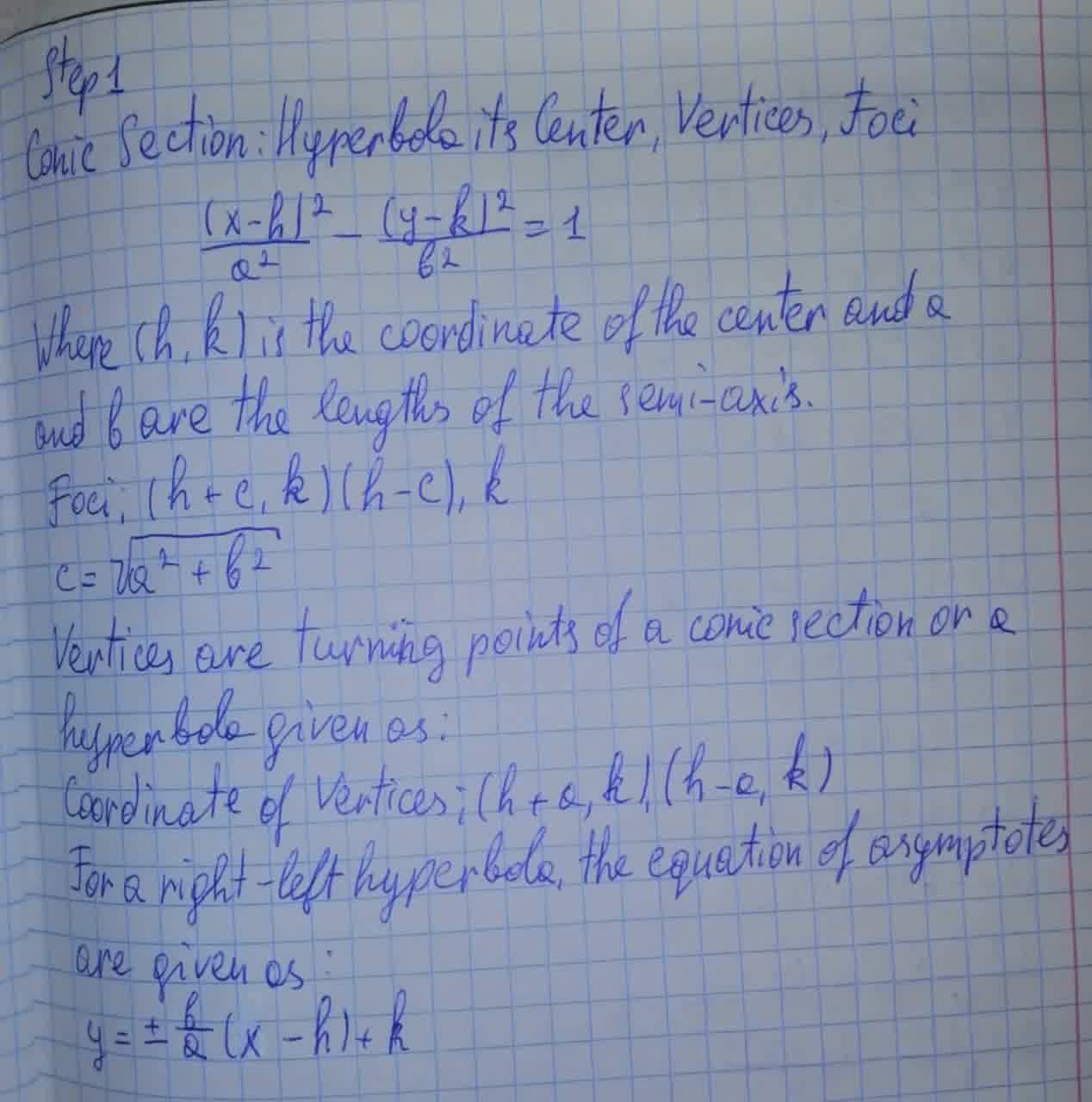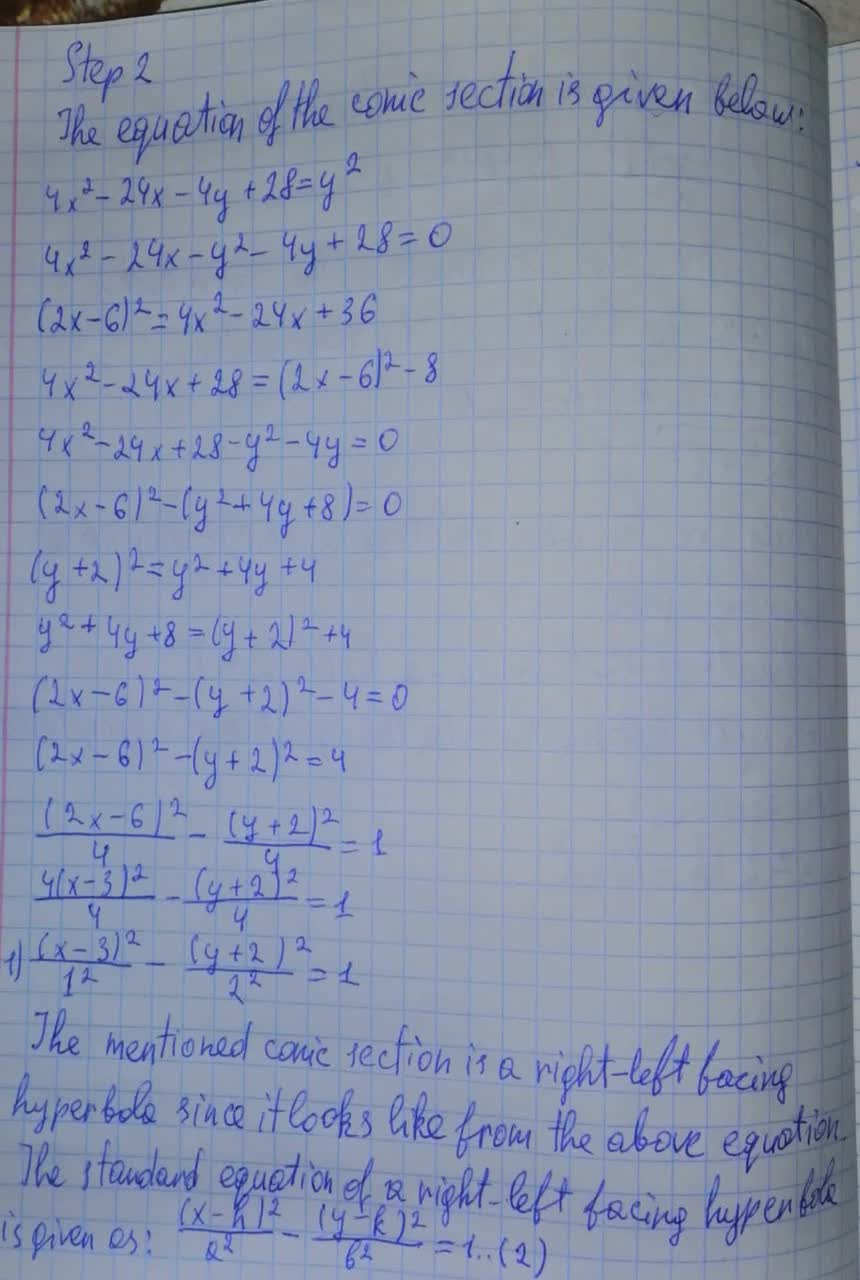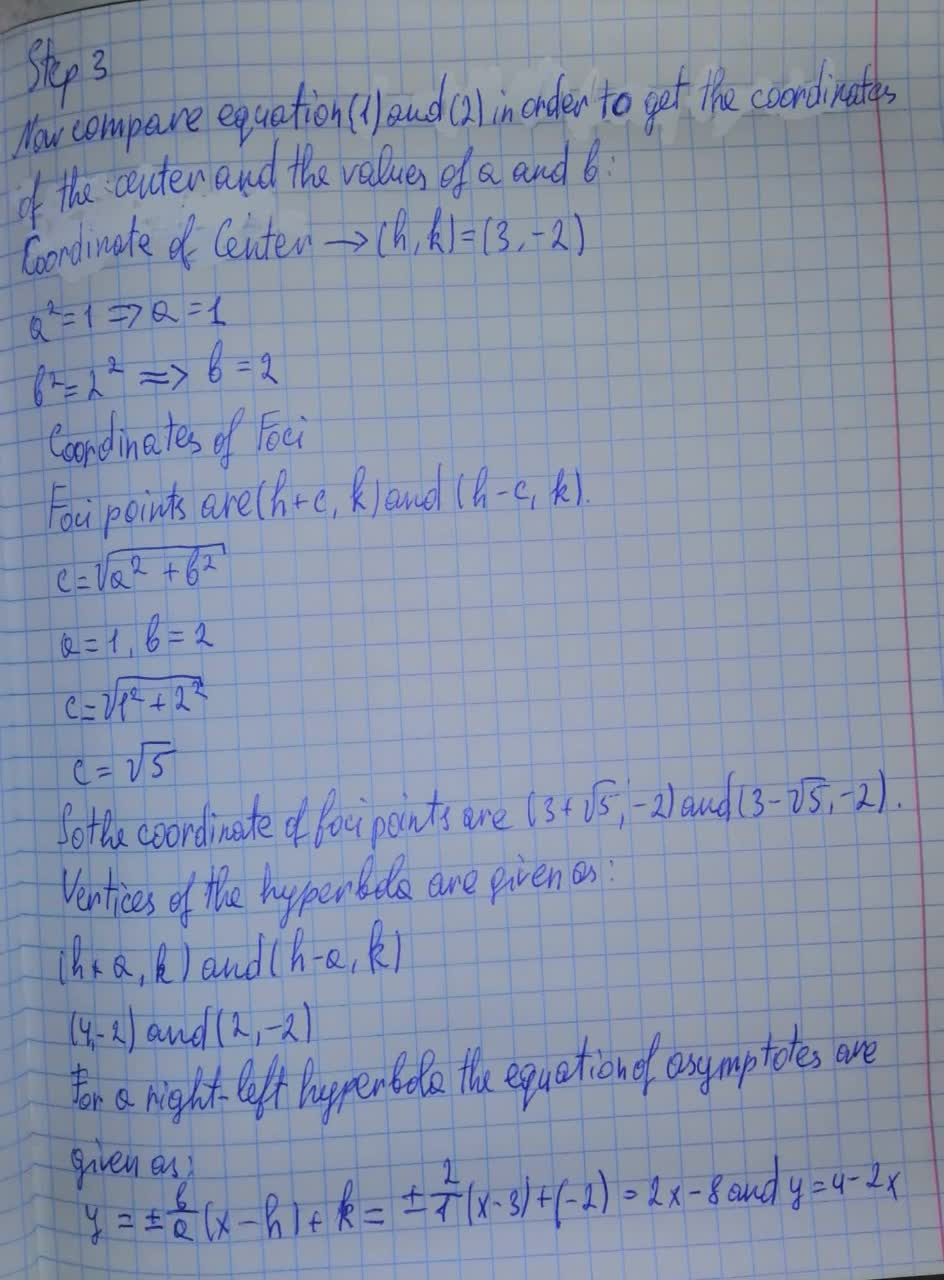Identify what type of conic section is given by the equation below and then find the center, foci, and vertices.Ernstfalld 2021-08-12 Answered
Identify what type of conic section is given by the equation below and then find the center, foci, and vertices. If it is a hyperbola, you should also find the asymptotes.
$$\displaystyle{4}{x}^{{2}}-{24}{x}-{4}{y}+{28}={y}^{{2}}$$• Questions are typically answered in as fast as 30 minutes

Solve your problem for the price of one coffee

• Math expert for every subject
• Pay only if we can solve itUsamah Prosser

Given that: